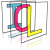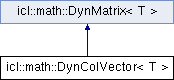Image Component Library (ICL)
icl::math::DynColVector< T > Struct Template Reference

Extension class for the DynMatrix<T> template, that restricts the the matrix column count to 'one'. More...

`#include <DynVector.h>`

Inheritance diagram for icl::math::DynColVector< T >:## Public Member Functions

DynColVector (const typename DynMatrix< T >::DynMatrixColumn &column)
creates a column vector from given matrix column More...

DynColVector ()
Default empty constructor creates a null-vector. More...

DynColVector (unsigned int dim, const T &initValue=0)
Creates a column vector with given dimension (and optional initialValue) More...

DynColVector (unsigned int dim, T *data, bool deepCopy=true)
Create a column vector with given data. More...

DynColVector (unsigned int dim, const T *data)
Creates column vector with given data pointer and dimsion (const version: deepCopy only) More...

DynColVector (const DynMatrix< T > &other)
Default copy constructor (the source matrix column count must be 'one') More...

DynColVector< T > & operator= (const DynMatrix< T > &other)
assignment operator (the rvalue's column count must be one) More...

void setBounds (unsigned int dim, bool holdContent=false, const T &initializer=0)

void setDim (unsigned int dim, bool holdContent=false, const T &initializer=0)Public Member Functions inherited from icl::math::DynMatrix< T >
DynMatrix (const DynMatrixColumn &column)
creates a column matrix from given column of other matrix More...

DynMatrix ()
Default empty constructor creates a null-matrix. More...

DynMatrix (unsigned int cols, unsigned int rows, const T &initValue=0)
Create a dyn matrix with given dimensions (and optional initialValue) More...

DynMatrix (unsigned int cols, unsigned int rows, T *data, bool deepCopy=true)
Create a matrix with given data. More...

DynMatrix (unsigned int cols, unsigned int rows, const T *data)
Create a matrix with given data (const version: deepCopy only) More...

DynMatrix (const DynMatrix &other)
Default copy constructor. More...

DynMatrix (const std::string &filename)
creates a new DynMatrix from given csv filename More...

void saveCSV (const std::string &filename)
writes the current matrix to a csv file More...

bool isNull () const
returns with this matrix has a valid data pointer More...

~DynMatrix ()
Destructor (deletes data if no wrapped shallowly) More...

DynMatrixoperator= (const DynMatrix &other)
Assignment operator (using deep/shallow-copy) More...

void setBounds (unsigned int cols, unsigned int rows, bool holdContent=false, const T &initializer=0)
resets matrix dimensions More...

bool isSimilar (const DynMatrix &other, T tollerance=T(0.0001)) const
tests weather a matrix is enough similar to another matrix More...

bool operator== (const DynMatrix &other) const
elementwise comparison (==) More...

bool operator!= (const DynMatrix &other) const
elementwise comparison (!=) More...

DynMatrix operator * (T f) const
Multiply elements with scalar. More...

DynMatrixmult (T f, DynMatrix &dst) const
Multiply elements with scalar (in source destination fashion) More...

DynMatrixoperator *= (T f)
Multiply elements with scalar (inplace) More...

DynMatrix operator/ (T f) const
Device elements by scalar. More...

DynMatrixoperator/= (T f)
Device elements by scalar (inplace) More...

DynMatrixmult (const DynMatrix &m, DynMatrix &dst) const
Matrix multiplication (in source destination fashion) [IPP-Supported]. More...

DynMatrixelementwise_mult (const DynMatrix &m, DynMatrix &dst) const
Elementwise matrix multiplication (in source destination fashion) [IPP-Supported]. More...

DynMatrix elementwise_mult (const DynMatrix &m) const
Elementwise matrix multiplication (without destination matrix) [IPP-Supported]. More...

DynMatrixelementwise_div (const DynMatrix &m, DynMatrix &dst) const
Elementwise division (in source destination fashion) [IPP-Supported]. More...

DynMatrix elementwise_div (const DynMatrix &m) const
Elementwise matrix multiplication (without destination matrix) [IPP-Supported]. More...

DynMatrix operator * (const DynMatrix &m) const
Essential matrix multiplication [IPP-Supported]. More...

DynMatrixoperator *= (const DynMatrix &m)
inplace matrix multiplication applying this = this*m [IPP-Supported] More...

DynMatrix operator/ (const DynMatrix &m) const
inplace matrix devision (calling this/m.inv()) [IPP-Supported] More...

DynMatrixoperator/= (const DynMatrix &m) const
inplace matrix devision (calling this/m.inv()) (inplace) More...

DynMatrix operator+ (const T &t) const
adds a scalar to each element More...

DynMatrix operator- (const T &t) const
substacts a scalar from each element More...

DynMatrixoperator+= (const T &t)
adds a scalar to each element (inplace) More...

DynMatrixoperator-= (const T &t)
substacts a scalar from each element (inplace) More...

DynMatrix operator+ (const DynMatrix &m) const

DynMatrix operator- (const DynMatrix &m) const
Matrix substraction. More...

DynMatrixoperator+= (const DynMatrix &m)

DynMatrixoperator-= (const DynMatrix &m)
Matrix substraction (inplace) More...

T & operator() (unsigned int col, unsigned int row)
element access operator (x,y)-access index begin 0! More...

const T & operator() (unsigned int col, unsigned int row) const
element access operator (x,y)-access index begin 0! (const) More...

T & at (unsigned int col, unsigned int row)
element access with index check More...

const T & at (unsigned int col, unsigned int row) const
element access with index check (const) More...

T & operator[] (unsigned int idx)

const T & operator[] (unsigned int idx) const

norm (double l=2) const
applies an L_l norm on the matrix elements (all elements are treated as vector) More...

sqrDistanceTo (const DynMatrix &other) const
returns the squared distance of the inner data vectors (linearly interpreted) (IPP accelerated) More...

distanceTo (const DynMatrix &other) const
returns the distance of the inner data vectors (linearly interpreted) (IPP accelerated) More...

unsigned int rows () const
height of the matrix (number of rows) More...

unsigned int cols () const
width of the matrix (number of columns) More...

T * data ()
internal data pointer More...

const T * data () const
internal data pointer (const) More...

unsigned int dim () const
matrix dimension (width*height) or (cols*rows) More...

int stride0 () const
returns sizeof (T)*dim() More...

int stride1 () const
returns sizeof(T)*cols() More...

int stride2 () const
returns sizeof (T) More...

DynMatrixoperator= (const DynMatrixColumn &col)

iterator begin ()
returns an iterator to the begin of internal data array More...

iterator end ()
returns an iterator to the end of internal data array More...

const_iterator begin () const
returns an iterator to the begin of internal data array (const) More...

const_iterator end () const
returns an iterator to the end of internal data array (const) More...

col_iterator col_begin (unsigned int col)
returns an iterator running through a certain matrix column More...

col_iterator col_end (unsigned int col)
returns an iterator end of a certain matrix column More...

const_col_iterator col_begin (unsigned int col) const
returns an iterator running through a certain matrix column (const) More...

const_col_iterator col_end (unsigned int col) const
returns an iterator end of a certain matrix column (const) More...

row_iterator row_begin (unsigned int row)
returns an iterator running through a certain matrix row More...

row_iterator row_end (unsigned int row)
returns an iterator of a certains row's end More...

const_row_iterator row_begin (unsigned int row) const
returns an iterator running through a certain matrix row (const) More...

const_row_iterator row_end (unsigned int row) const
returns an iterator of a certains row's end (const) More...

DynMatrix row (int row)
Extracts a shallow copied matrix row. More...

const DynMatrix row (int row) const
Extracts a shallow copied matrix row (const) More...

DynMatrixColumn col (int col)
Extracts a shallow copied matrix column. More...

const DynMatrixColumn col (int col) const

void decompose_QR (DynMatrix &Q, DynMatrix &R) const
applies QR-decomposition using stabilized Gram-Schmidt orthonormalization (only for icl32f and icl64f) More...

void decompose_RQ (DynMatrix &R, DynMatrix &Q) const
applies RQ-decomposition (by exploiting implemnetation of QR-decomposition) (only for icl32f, and icl64f) More...

void decompose_LU (DynMatrix &L, DynMatrix &U, T zeroThreshold=T(1E-16)) const
applies LU-decomposition (without using partial pivoting) (only for icl32f and icl64f) More...

DynMatrix solve_upper_triangular (const DynMatrix &b) const
solves Mx=b for M=*this (only if M is a squared upper triangular matrix) (only for icl32f and icl64f) More...

DynMatrix solve_lower_triangular (const DynMatrix &b) const
solves Mx=b for M=*this (only if M is a squared lower triangular matrix) (only for icl32f and icl64f) More...

DynMatrix solve (const DynMatrix &b, const std::string &method="lu", T zeroThreshold=T(1E-16))
solves Mx=b for M=*this (only for icl32f and icl64f) More...

DynMatrix inv () const
invert the matrix (only for icl32f and icl64f) More...

void eigen (DynMatrix &eigenvectors, DynMatrix &eigenvalues) const
Extracts the matrix's eigenvalues and eigenvectors. More...

void svd (DynMatrix &U, DynMatrix &S, DynMatrix &V) const
Computes Singular Value Decomposition of a matrix - decomposes A into USV'. More...

DynMatrix pinv (bool useSVD=false, T zeroThreshold=T(1E-16)) const
calculates the Moore-Penrose pseudo-inverse (only implemented for icl32f and icl64f) More...

DynMatrix big_matrix_pinv (T zeroThreshold=T(1E-16)) const
calculates the Moore-Penrose pseudo-inverse (specialized for big matrices) More...

DynMatrix big_matrix_pinv (T zeroThreshold, GESDD gesdd, CBLAS_GEMM cblas_gemm) const

det () const
matrix determinant (only for icl32f and icl64f) More...

DynMatrix transp () const
matrix transposed More...

const DynMatrix< T > shallowTransposed () const
returns a shallow transposed copy of this matrix (dimensions are swapped, data is not re-aranged) (const) More...

const DynMatrix< T > shallowTransposed ()
returns a shallow transposed copy of this matrix (dimensions are swapped, data is not re-aranged) More...

void reshape (int newCols, int newRows)
resets the matrix dimensions without changing the content More...

element_wise_inner_product (const DynMatrix< T > &other) const
inner product of data pointers (not matrix-mulitiplication) More...

DynMatrix< T > dot (const DynMatrix< T > &M) const
returns the inner product of two matrices (i.e. dot-product) More...

DynMatrix< T > diag () const
returns diagonal-elements as column-vector More...

trace () const
computes the sum of all diagonal elements More...

cond (const double p=2) const
computes the condition of a matrix More...

T * set_data (T *newData)
sets new data internally and returns old data pointer (for experts only!) More...Public Types inherited from icl::math::DynMatrix< T >
typedef T * iterator
default iterator type (just a data-pointer) More...

typedef const T * const_iterator
dafault const_iterator type (just a data-pointer) More...

typedef T * row_iterator
comples row_iterator type More...

typedef const T * const_row_iterator
complex const_row_iterator type More...

typedef const col_iterator const_col_iterator
const column iterator typedef More...

typedef void(* GESDD) (const char *, const int *, const int *, T *, const int *, T *, T *, const int *, T *, const int *, T *, const int *, int *, int *)

typedef void(* CBLAS_GEMM) (CBLAS_ORDER, CBLAS_TRANSPOSE, CBLAS_TRANSPOSE, int, int, int, T, const T *, int, const T *, int, T, T *, int)Static Public Member Functions inherited from icl::math::DynMatrix< T >
static DynMatrix< T > loadCSV (const std::string &filename)
loads a dynmatrix from given CSV file More...

static DynMatrix< T > cross (const DynMatrix< T > &x, const DynMatrix< T > &y)
computes the cross product More...

static DynMatrix id (unsigned int dim)
creates a dim-D identity Matrix More...

## Detailed Description

### template<class T> struct icl::math::DynColVector< T >

Extension class for the DynMatrix<T> template, that restricts the the matrix column count to 'one'.

## ◆ DynColVector() [1/6]

template<class T>
 icl::math::DynColVector< T >::DynColVector ( const typename DynMatrix< T >::DynMatrixColumn & column )
inline

creates a column vector from given matrix column

## ◆ DynColVector() [2/6]

template<class T>
 icl::math::DynColVector< T >::DynColVector ( )
inline

Default empty constructor creates a null-vector.

## ◆ DynColVector() [3/6]

template<class T>
 icl::math::DynColVector< T >::DynColVector ( unsigned int dim, const T & initValue = `0` )
inline

Creates a column vector with given dimension (and optional initialValue)

## ◆ DynColVector() [4/6]

template<class T>
 icl::math::DynColVector< T >::DynColVector ( unsigned int dim, T * data, bool deepCopy = `true` )
inline

Create a column vector with given data.

Data can be wrapped deeply or shallowly. If the latter is true, given data pointer will not be released in the destructor, i.e. the data ownership is not passed to the DynColumnVector instance

## ◆ DynColVector() [5/6]

template<class T>
 icl::math::DynColVector< T >::DynColVector ( unsigned int dim, const T * data )
inline

Creates column vector with given data pointer and dimsion (const version: deepCopy only)

## ◆ DynColVector() [6/6]

template<class T>
 icl::math::DynColVector< T >::DynColVector ( const DynMatrix< T > & other )
inline

Default copy constructor (the source matrix column count must be 'one')

## ◆ operator=()

template<class T>
 DynColVector& icl::math::DynColVector< T >::operator= ( const DynMatrix< T > & other )
inline

assignment operator (the rvalue's column count must be one)

## ◆ setBounds()

template<class T>
 void icl::math::DynColVector< T >::setBounds ( unsigned int dim, bool holdContent = `false`, const T & initializer = `0` )
inline

overwrites setBounds from the parent matrix class to prevent the vector from being resized to matrix bounds

## ◆ setDim()

template<class T>
 void icl::math::DynColVector< T >::setDim ( unsigned int dim, bool holdContent = `false`, const T & initializer = `0` )
inline